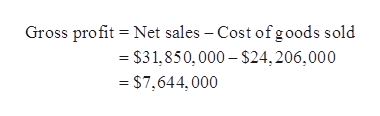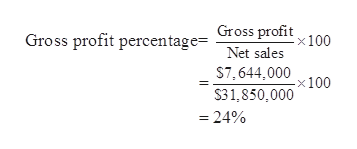# During the current year, merchandise is sold for \$31,850,000. The cost of the merchandise sold is \$24,206,000.a. What is the amount of the gross profit?b. Compute the gross profit percentage (gross profit divided by sales).c. Will the income statement necessarily report a net income? Explain.

Question
1 views

During the current year, merchandise is sold for \$31,850,000. The cost of the merchandise sold is \$24,206,000.
a. What is the amount of the gross profit?
b. Compute the gross profit percentage (gross profit divided by sales).
c. Will the income statement necessarily report a net income? Explain.

check_circle

Step 1

Gross Profit:

Gross profit represents the revenue after the deduction of cost of goods sold from the net sales of a business.

Step 2

a.

Ascertain the amount of gross profit.

Hence, the amount of gross profit is \$7,644,000.help_outlineImage TranscriptioncloseGross profit = Net sales – Cost of goods sold = \$31,850, 000 – \$24, 206,000 = \$7,644, 000 fullscreen
Step 3

b.

Compute the gross profit percentage.

Hence, the gross pr...help_outlineImage TranscriptioncloseGross profit x100 Gross profit percentage= Net sales S7, 644,000 -x100 S31,850,000 = 24% fullscreen

### Want to see the full answer?

See Solution

#### Want to see this answer and more?

Solutions are written by subject experts who are available 24/7. Questions are typically answered within 1 hour.*

See Solution
*Response times may vary by subject and question.
Tagged in